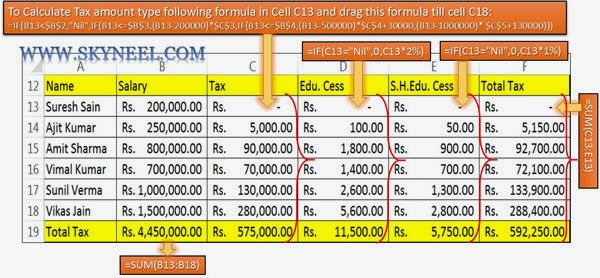# Income Tax Calculation Formula With IF Statement in Excel

compute his tax liabilities for salaried individuals by Income Tax Calculation Formula With IF Statement and Nested IF Function in MS Excel.

You can easily compute his tax liabilities for salaried individuals with the help of Income Tax Calculation Formula With IF Statement in MS Excel with the help of Nested IF Function. “IF” and “Nested IF” functions are very popular functions in “Logical functions” category in MS Excel. Nested IF function is used when another IF function is assigned inward the first IF function in order to check extra situations.

For example, IF you want to calculate income tax, education cess and secondary higher education-cess as per slab for income tax of AY 2013-14, the slab rate of income tax is given below: Individual resident whose age is lower than sixty years (That means whose date of birth is on or after the 1st Apr. , 1953) or any Non Resident Indian / Hindu UN-Divided Family / Association of Person / Body of Individuals / Artificial Judicial Person Income-tax: Calculate Liability for Tax for AY 2013-14

Additional Tax : “No Surcharge”Education Cess: 2% of the Income Tax Amount.Secondary Higher Education Cess: 1% of the Income Tax Amount.

To calculate income tax as per given table you have to take following steps in excel sheet:

 S. No. Income Slabs Income Tax Rate 1. Where the total earning is not greater than equal to Rs. 200000/-. – 2. Where the total earning is greater than Rs. 2,00,000/- but less than Rs. (500000) five lakhs. 10% of the sum of earning which greater than Rs. 2,00,000/- 3. Where the total earning is greater than Rs. (5,00,000) lakhs but less than Rs. (1000000) lakhs. Rs. (30,000) Thirty Thousand + 20% of the whole amount which is the whole earning larger than Rs. (500000) five lakhs/-. 4. Where the total income greater than Rs. (1000000) Ten Lakhs. Rs. (130,000) One Lakh and Thirty Thousand + 30% of the whole amount which is the whole earning larger than Rs.  (1000000) Ten Lakhs.

## Income Tax Calculation Formula With IF Statement in ExcelStep 1: To calculate the income tax with the help of IF function you have to prepare following sheet in excel.

Step 2: To calculate income tax amount you have to type following given formula in cell C13  =IF(B13<\$B\$2,”Nil”,IF(B13<=\$B\$3,(B13-200000)*\$C\$3,IF(B13<=\$B\$4,(B13-500000)*\$C\$4+30000,(B13-1000000)*\$C\$5+130000))).

Step 3: After calculate income tax amount you have to calculate education cess @ 2% on income tax in cell D13:  =IF(C13=”Nil”,0,C13*2%).

You have to calculate Secondary Higher Education Cess @ 1% on income tax amount as per the given formula in cell E13 :  =IF(C13=”Nil”,0,C13*1%).

Now calculate total of tax amount in cell F13:    =SUM(C13:E13).

Copy formula of income tax, edu. cess and secondary higher education cess by drag and drop method in given range C14:F18.I hope you like this Income Tax Calculation Formula With IF Statement guide. This post is useful to calculate income tax every year, but you have to change income tax slab as per given income tax department. Give your important suggestion regarding this post in comment box.and also share this article with your friends to show your knowledge in excel. Thanks.

1. I WANT IF FORMULA FOR IT CALCULATION FOR THE PERCENTAGE OF 5%,10%,15%,20% AND 25% FOR THE RANGES FOR UP TO 2.5 LAKHS 0, 2.5 TO 5.0 LAKHS 5%, FROM 5.0 TO 7.5 LAKHS IS 10%, FROM 7.5 TO 10.0 LAKHS IS 15%, FROM 10.0 TO 12.5LAKHS IS 20% AND 15.5 TO 15.0 LAKHS IS 25% AND ABOVE 15.0 LAKHS IS 30%. PLEASE GIVE FORMULA FOR THIS.

1. =ROUND(IF(BR112<250000,0,IF(BR112<500000,(BR112-250000)*0.05,IF(BR112<750000,(BR112-500000)*0.1+12500,IF(BR112<1000000,(BR112-750000)*0.15+37500,IF(BR112<1250000,(BR112-1000000)*0.2+75000,IF(BR1121500000,(BR112-1500000)*0.3+187500))))))),0)

2. Dear Sir this is very usefull to us but one problem is there, that is as per your formula Income Tax calculated only10% rest of 20% and 30% is calculated wrong. Kindly clarify it.

3. what if the tax slab for married and unmarried person are different further if tax rate slab for female staff and male staff are different .
for example
Income Tax Remarks
First 200000 1% Married
First 150000 1% Unmarried

4. Sir
If we have not deducted TDS from salary as his salary is under taxable limit are we need to give him Form-16 compulsory ?

5. Sir,when I am calculating tax liability then for the 1st employee in our list is OK. But when I drag the formula for our subsequent employee then we getting the tax liability is wrong. Please let me inform.